# Computer Architecture and Assembly Language Practical Session 12

• Slides: 12Computer Architecture and Assembly Language Practical Session 12 Memory access Performance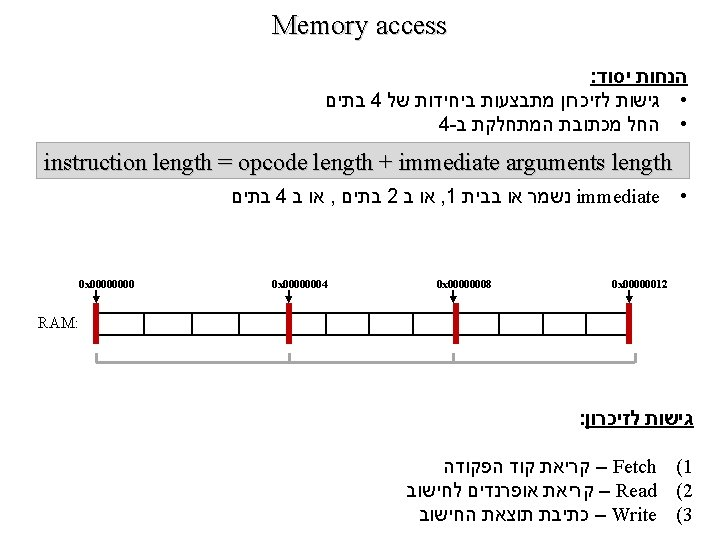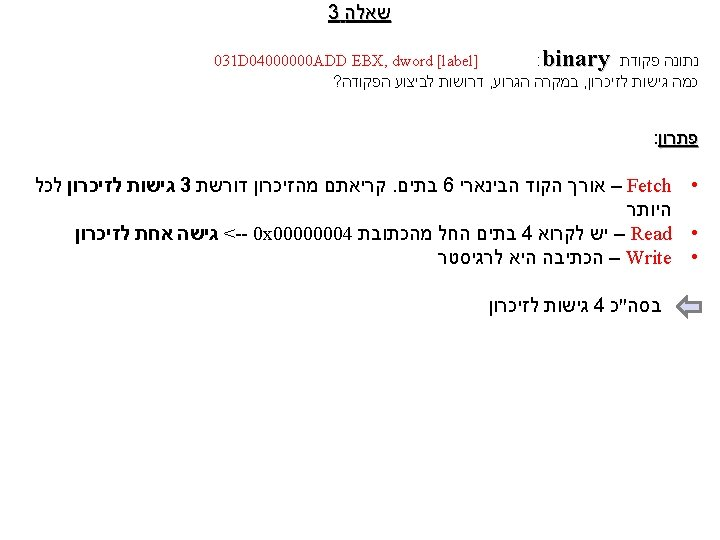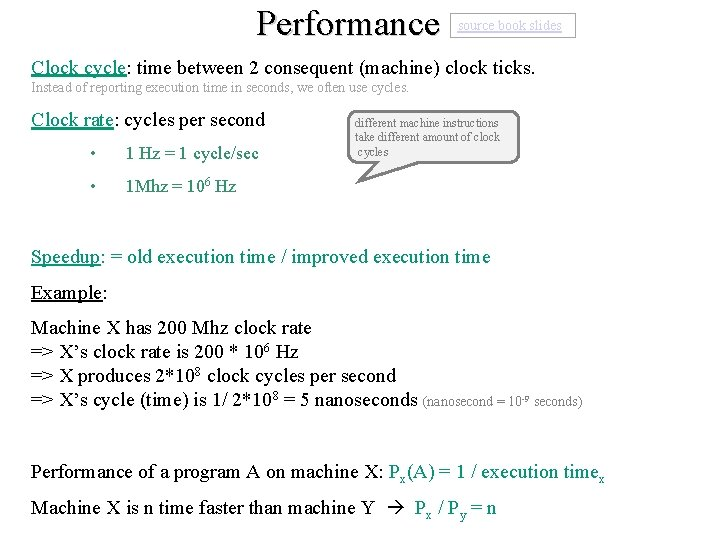Performance source book slides Clock cycle: time between 2 consequent (machine) clock ticks. Instead of reporting execution time in seconds, we often use cycles. Clock rate: cycles per second • 1 Hz = 1 cycle/sec • 1 Mhz = 106 Hz different machine instructions take different amount of clock cycles Speedup: = old execution time / improved execution time Example: Machine X has 200 Mhz clock rate => X’s clock rate is 200 * 106 Hz => X produces 2*108 clock cycles per second => X’s cycle (time) is 1/ 2*108 = 5 nanoseconds (nanosecond = 10 -9 seconds) Performance of a program A on machine X: Px(A) = 1 / execution timex Machine X is n time faster than machine Y Px / Py = n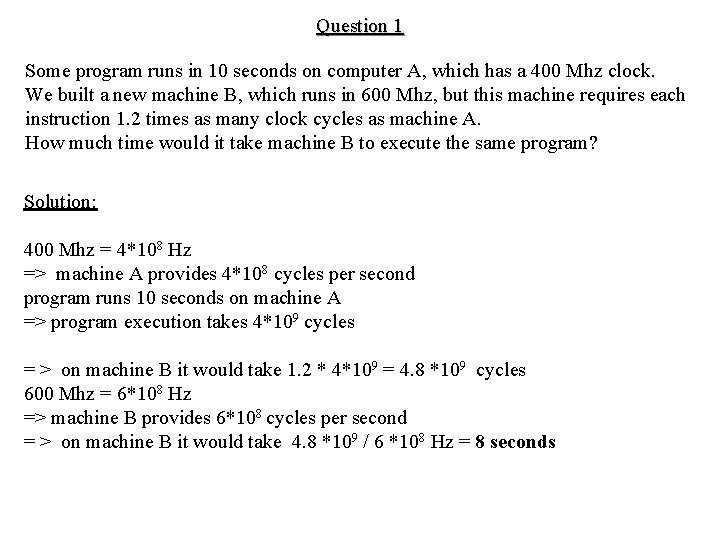Question 1 Some program runs in 10 seconds on computer A, which has a 400 Mhz clock. We built a new machine B, which runs in 600 Mhz, but this machine requires each instruction 1. 2 times as many clock cycles as machine A. How much time would it take machine B to execute the same program? Solution: 400 Mhz = 4*108 Hz => machine A provides 4*108 cycles per second program runs 10 seconds on machine A => program execution takes 4*109 cycles = > on machine B it would take 1. 2 * 4*109 = 4. 8 *109 cycles 600 Mhz = 6*108 Hz => machine B provides 6*108 cycles per second = > on machine B it would take 4. 8 *109 / 6 *108 Hz = 8 seconds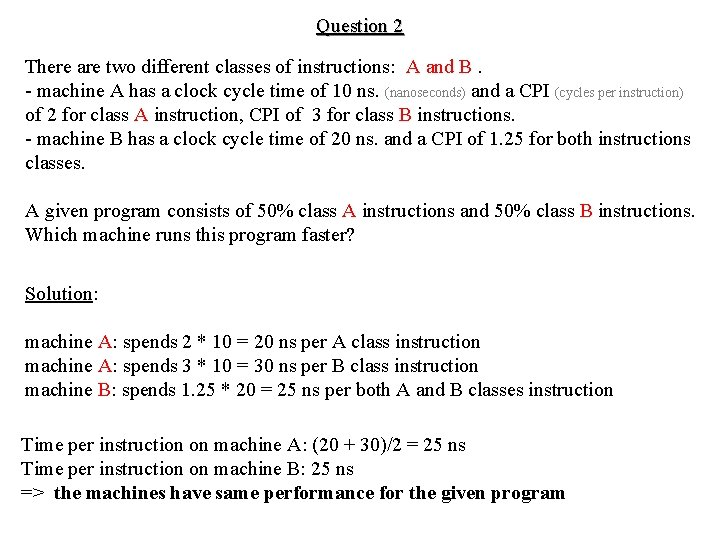Question 2 There are two different classes of instructions: A and B. - machine A has a clock cycle time of 10 ns. (nanoseconds) and a CPI (cycles per instruction) of 2 for class A instruction, CPI of 3 for class B instructions. - machine B has a clock cycle time of 20 ns. and a CPI of 1. 25 for both instructions classes. A given program consists of 50% class A instructions and 50% class B instructions. Which machine runs this program faster? Solution: machine A: spends 2 * 10 = 20 ns per A class instruction machine A: spends 3 * 10 = 30 ns per B class instruction machine B: spends 1. 25 * 20 = 25 ns per both A and B classes instruction Time per instruction on machine A: (20 + 30)/2 = 25 ns Time per instruction on machine B: 25 ns => the machines have same performance for the given program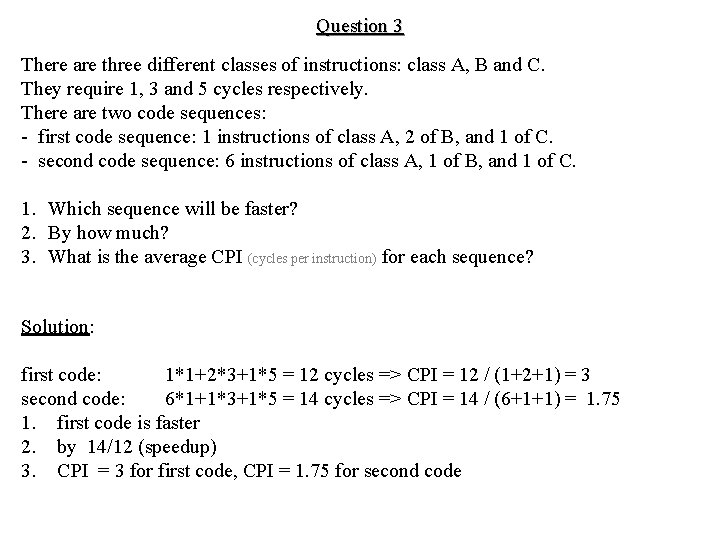Question 3 There are three different classes of instructions: class A, B and C. They require 1, 3 and 5 cycles respectively. There are two code sequences: - first code sequence: 1 instructions of class A, 2 of B, and 1 of C. - second code sequence: 6 instructions of class A, 1 of B, and 1 of C. 1. Which sequence will be faster? 2. By how much? 3. What is the average CPI (cycles per instruction) for each sequence? Solution: first code: 1*1+2*3+1*5 = 12 cycles => CPI = 12 / (1+2+1) = 3 second code: 6*1+1*3+1*5 = 14 cycles => CPI = 14 / (6+1+1) = 1. 75 1. first code is faster 2. by 14/12 (speedup) 3. CPI = 3 for first code, CPI = 1. 75 for second code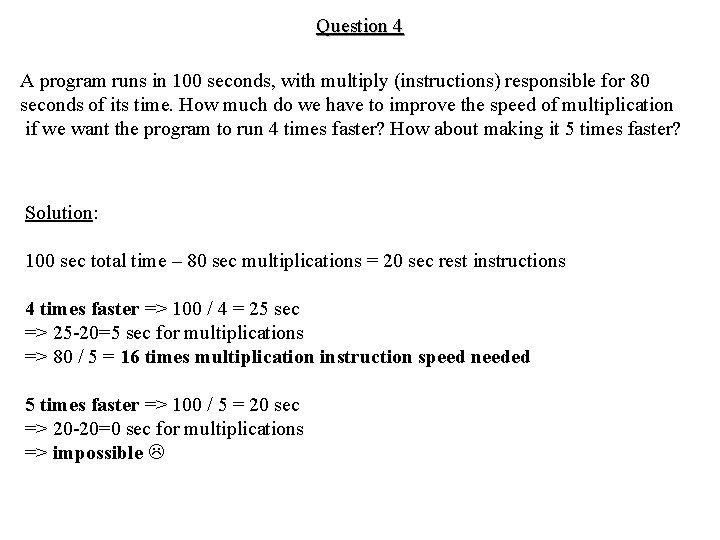Question 4 A program runs in 100 seconds, with multiply (instructions) responsible for 80 seconds of its time. How much do we have to improve the speed of multiplication if we want the program to run 4 times faster? How about making it 5 times faster? Solution: 100 sec total time – 80 sec multiplications = 20 sec rest instructions 4 times faster => 100 / 4 = 25 sec => 25 -20=5 sec for multiplications => 80 / 5 = 16 times multiplication instruction speed needed 5 times faster => 100 / 5 = 20 sec => 20 -20=0 sec for multiplications => impossibleQuestion 5 Given a code runs for 20 sec, 14 sec for floating point instructions, and 6 sec for rest. A code consists of 70% floating-point instructions. We improved floating-point instructions run 7 times faster, but caused rest of the instructions run double the time. What will the speedup be? Solution: execution time after improvement = 6*2 sec+ 14 sec / 7 = 12+2 = 14 sec => speedup = 20 / 14